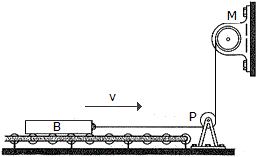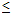# Engineering Mechanics - KOP: Force and Acceleration - Discussion

### Discussion :: KOP: Force and Acceleration - General Questions (Q.No.3)

3.The 300-kg bar B, originally at rest, is being towed over a series of small rollers. Computer the force in the cable when t = 5s, if the motor M is drawing in the cable for a short time at a rate of v = (0.4t2) m/s, where t is in seconds (0t6 s). How far does the bar move in 5 s? Neglect the mass of the cable, pulley P, and the rollers.

 [A]. T = 5.00 kN, s = 0.300 m [B]. T = 1.200 kN, s = 1.25 m [C]. T = 5.00 kN, s = 4.00 m [D]. T = 1.200 kN, s = 16.67 m

Explanation:

No answer description available for this question.